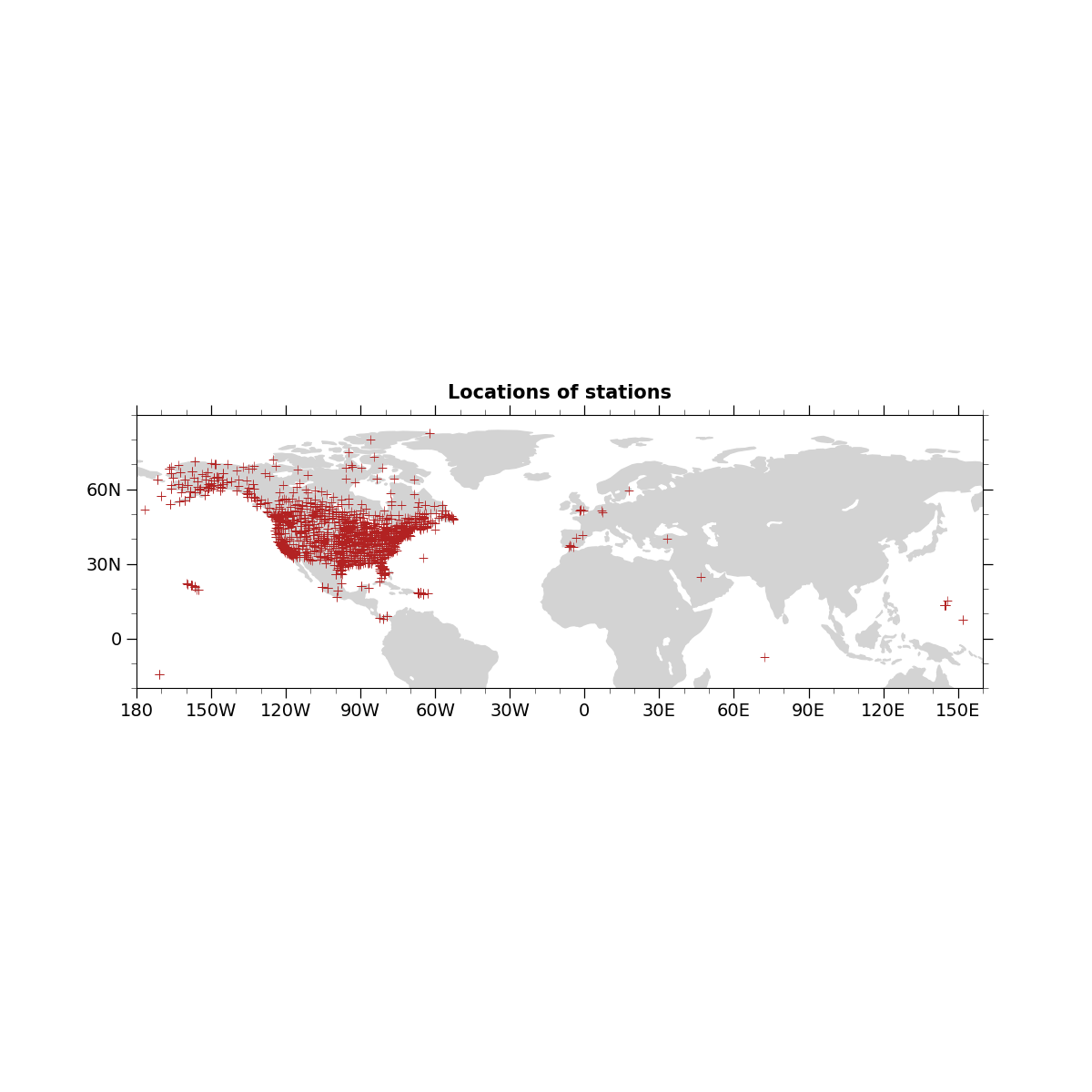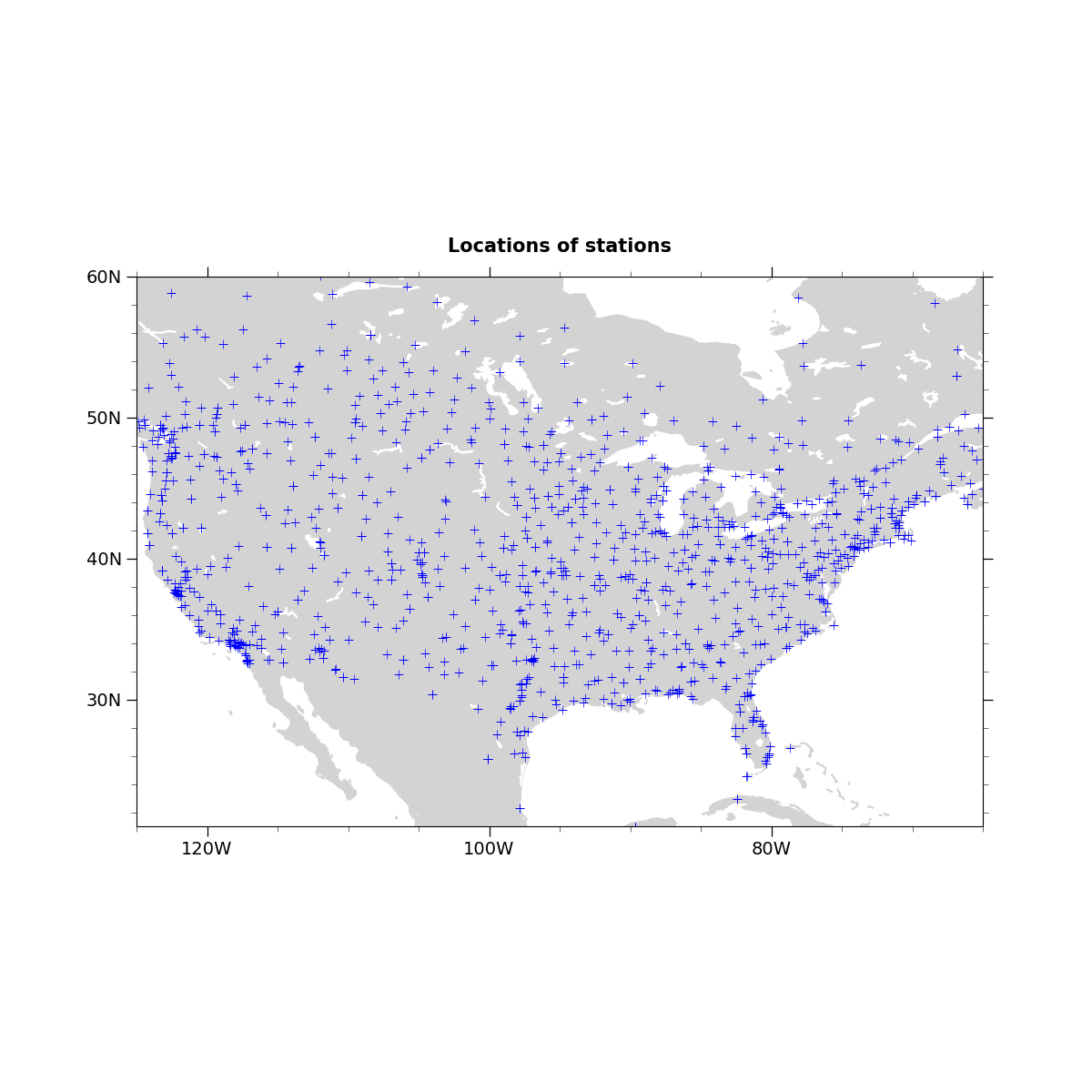# NCL_scatter_3.py#

This script illustrates the following concepts:
• Drawing a scatter plot over a map

• Choosing marker color, size, and style

• Drawing markers on a map indicating the locations of station data

See following URLs to see the reproduced NCL plot & script:

Import packages:

```import xarray as xr
import cartopy.crs as ccrs
import cartopy.feature as cfeature
from cartopy.mpl.gridliner import LongitudeFormatter, LatitudeFormatter
import matplotlib.pyplot as plt

import geocat.viz as gv
import geocat.datafiles as gdf
```

Open a netCDF data file using xarray default engine and load the data into xarrays

```ds = xr.open_dataset(gdf.get("netcdf_files/95031800_sao.cdf"),
decode_times=False)
lat = ds.lat.isel()
lon = ds.lon.isel()
```

Plot

```def Plots(xlim, ylim, xtic, ytic, xminor, yminor, size, color):
"""Creates plot using user specified variables.

Parameters
----------
xext : 'tuple'
Inclusive extent of projection in format (xstart, xend) with values between -180
and 180.
yext : 'tuple'
Inclusive extent of projection in format (ystart, yend) with values between -90
and 90.
xtic : 'int'
Step number of major x tick label instances in format of number between each tick.
This is passed to range() as the 'step' argument.
ytic : 'int'
Step number of major y tick label instances in format of number between each tick.
This is passed to range() as the 'step' argument.
xminor : 'int'
Exclusive number of minor ticks between each major x-axis tick mark
yminor : 'int'
Exclusive number of minor ticks between each major y-axis tick mark
size : 'int'
Size of marker being used in format of font size number.
color : 'str'
Matplotlib color of marker being used in format 'color'.
"""

# Generate figure (set its size (width, height) in inches) and axes using Cartopy projection
plt.figure(figsize=(12, 12))

# Generate axes using Cartopy
ax = plt.axes(projection=ccrs.PlateCarree())

# Use geocat.viz.util convenience function to add minor and major tick lines
x_minor_per_major=xminor,
y_minor_per_major=yminor,
labelsize=14)

# Use geocat.viz.util convenience function to make plots look like NCL plots by using latitude, longitude tick labels

# Use geocat.viz.util convenience function to set axes limits & tick values without calling several matplotlib functions
gv.set_axes_limits_and_ticks(ax,
xlim=xlim,
ylim=ylim,
xticks=range(-180, 180, xtic),
yticks=range(-90, 90, ytic))

# Remove the degree symbol from tick labels
ax.yaxis.set_major_formatter(LatitudeFormatter(degree_symbol=''))
ax.xaxis.set_major_formatter(LongitudeFormatter(degree_symbol=''))

edgecolor='lightgray',
facecolor='lightgray',
zorder=0)
edgecolor='white',
facecolor='white',
zorder=0)

# Scatter-plot the location data on the map
plt.scatter(lon, lat, s=size, c=color, marker='+', linewidth=0.5, zorder=1)

plt.title("Locations of stations",
loc="center",
y=1.03,
size=15,
fontweight="bold")

plt.show()

Plots((-180, 160), (-20, 90), 30, 30, 3, 3, 50, 'firebrick')
Plots((-125, -65), (21, 60), 20, 10, 4, 5, 50, 'blue')
```
••Total running time of the script: ( 0 minutes 0.793 seconds)

Gallery generated by Sphinx-Gallery